Search:

Self Service

Step 1. The Cross

Step 2. F2L

Step 3. OLL

Step 4. PLL

This is full CFOP (or Fridrich) method. 'CFOP' refers to the steps involved - Cross, F2L, OLL and PLL. This involves remembering a lot of algorithms to cope with different situations, so I use some 2-look OLL and PLL to reduce the amount of algorithms I need to know.

## Step 1 - The Cross

This step is the same as all the other methods - forming a cross on the first layer to get this:However, there is one key difference: it is done on the bottom layer. This means that you can't always see what is happening with the cross, which sounds like a good way to just make solving the cube more difficult. The reason for doing the cross on the bottom layer is that you then don't have to turn the cube over when starting the second layer. This saves time not only by not physically moving the cube, but whilst you are completing the cross you can be looking ahead for your first move of F2L.

At this stage, a lot of people still find it quite difficult to intuitively manipulate the cube. This means that doing the cross on the bottom is difficult, as they have come to rely on algorithms for moving pieces that suddenly don't work as everything is upside-down. It is difficult to teach intuition, but through practice it will eventually just click. Don't be disheartened if doing the cube on the bottom takes many times longer than doing it on the top - if you're doing it at all you're improving. Also, don't be afraid to be move on in the advanced method while not being able to do the cross on the bottom. I have found that F2L (the next section) hugely helps people to understand how to move cubies to where they want them, a skill that they later use for the cross. You may never even go back to the cross, and continue doing it on the top. This is fine for most people, but if you really want to be pushing your time down to less than 20 seconds, you will need to learn at some point.

All of that being said, I will give you some situations to look out for whilst doing the cross on the bottom. Some of them may seem obvious, but they are there for people who need them.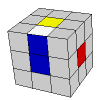F2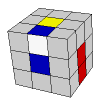U' R' F R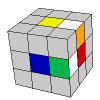F R2 D2

The first two cases here are quite similar to those given in the beginner method for the first cross on the top layer. They are quite simple, and you should be able to deal with them with relative ease. The third case is a little more complicated. You should, by now, be able to immediately recall that green is opposite blue and orange is opposite red. So instead of putting the white-orange edge piece where it needs to go and then the white-green edge piece, they can both be put in the opposite locations, i.e. the white-orange piece in the white-red location and the white-green piece in the white-blue location. After a simple D2, both are then in the correct place.

If you can comfortably form the cross on the bottom layer, your work is still not over. Now you need to start practising doing it efficiently. This means looking ahead, i.e. thinking about what you are going to do next when you've completed whatever it is you are doing. Again, this is not something that is easily taught. Different people will solve the same cube in different ways, and will likely disagree on the best or most efficient way of doing something. As you practise, you will get quicker and more confident with the cube until you too have your own version of the best way.

## Step 2 - F2L

This step involves solving the first layer corners and second layer at the same time, to get this: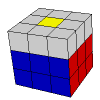To see all of the cases and what to do for each, go here.

In this situation, where the red-blue corner is to be done: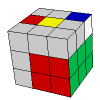You might do something like y' U R U' R' U y' R' U' R, as your natural instinct will likely be to use your right hand. Instead, L' U L U L' U' L works a lot better, as there is no slow rotating of the cube to be done.

There are some situations that you should just know the algorithm for: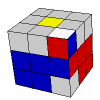(R U R' U') * 3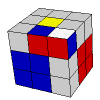(R U R' U') U' (R U R' U') (R U R')y' (R' U' R U) U (R' U' R U) (R' U' R)

## Step 3 - OLL

This step involves orienting all of the last layer cubies so the yellow face is complete, like this: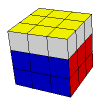Here are all of the OLL algorithms, in what I think is a logical order:
If you need help with notation, go here.

### [-] CrossR' U2 R U R' U R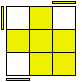L' U R U' L U R'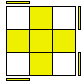L U' R' U L' U R U R' U R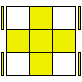R U R' U R U' R' U R U2 R'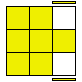R' F' L F R F' L' F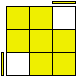R' F' L' F R F' L F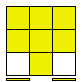R2 D R' U2 R D' R' U2 R'

### [-] Dot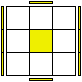R U B' l U l2' x' U' R' F R F'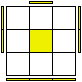R' F R F' U2 R' F R y' R2 U2 R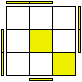y L' R2 B R' B L U2' L' B M'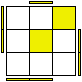R' U2 x R' U R U' y R' U' R' U R' F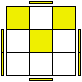R' U2 F R U R' U' y' R2 U2 x' R U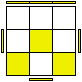R' U2 F R U R' U' y' R2 U2 x' R U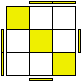R U R' U R' F R F' U2 R' F R F'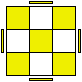M' U2 M U2 M' U M U2 M' U2 M

### [-] All Corners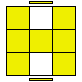L' R U R' U' L R' F R F'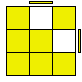M' U' M U2' M' U' M

### [-] Lines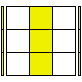R U' y R2 D R' U2 R D' R2 d R'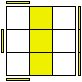R' U' y L' U L' y' L F L' F R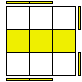F U R U' R' U R U' R' F'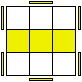L' B' L U' R' U R U' R' U R L' B L

### [-] Big Ls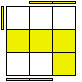R' F R U R' F' R y L U' L'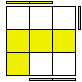L F' L' U' L F L' y' R' U R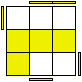R B R' L U L' U' R B' R'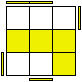L' B' L R' U' R U L' B L

### [-] Ts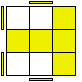F R U R' U' F'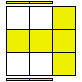R U R' U' R' F R F'

### [-] Zs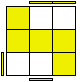R' F R U R' U' y L' d R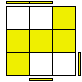L F' L' U' L F d R d' L'

### [-] Cs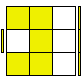R U x' R U' R' U x U' R'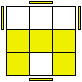R U R' U' x D' R' U R E'

### [-] Little Ls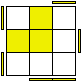F R U R' U' R U R' U' F'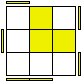F R U R' U' R U R' U' F'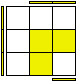L U' y' R' U2' R' U R U' R U2 R d' L'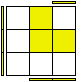R' F R' F' R2 U2 x' U' R U R'R' F R F' U2 R2 y R' F' R F'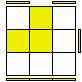L F' L' F U2 L2 y' L F L' F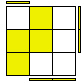L F R' F R F2 L'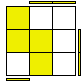L' B' L U' R' U R L' B L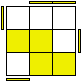U2 r R2' U' R U' R' U2 R U' M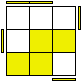U2 l' L2 U L' U L U2 L' U M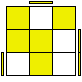x' U' R U' R2' F x R U R' U' R B2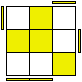R' U' R y' x' R U' R' F R U R'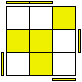R U R' y R' F R U' R' F' R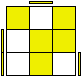R2' U R' B R U' R2' U l U l'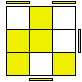U' R U2' R' U' R U' R2 y' R' U' R U B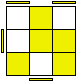U' R' U2 R U R' U R2 y R U R' U' F'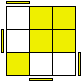R' U2 l R U' R' U l' U2 R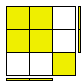F R' F' R U R U' R'

### [-] Ps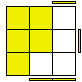L d R' d' L' U L F L'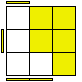R' d' L d R U' R' F' R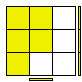F U R U' R' F'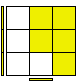F' U' L' U L F

### [-] Ws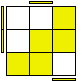L U L' U L U' L' U' y2' R' F R F'R' U' R U' R' U R U y F R' F' R

## Step 4 - PLL

This step involves permuting all of the last layer cubies so the cube is complete, like this: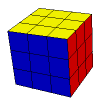Here are all of the PLL case algorithms, again in what I think is a logical order: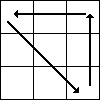x R2 D2 R U R' D2 R U' R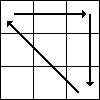x z' R2 U2 R' D' R U2 R' D R'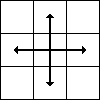M2 U M2 U2 M2 U M2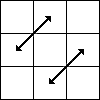R' U' R2 U R U R' U' R U R U' R U' R'R' U R' U' R' U' R' U R U R2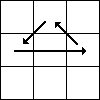R2 U' R' U' R U R U R U' R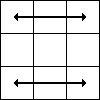R' F' L' F R F' L F R' F' L F R F' L' F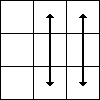U' R' U R U' R2 F' U' F U R F R' F' R2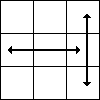R U R' U' R' F R2 U' R' U' R U R' F'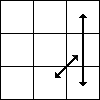R U R' F' R U R' U' R' F R2 U' R' U'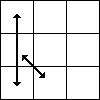L' U' L F L' U' L U L F' L2 U L U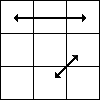R' U2 R U2 R' F R U R' U' R' F' R2 U'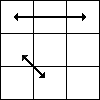L U2 L' U2 L F L' U' L U L F L2 U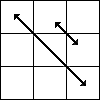R' U R' U' B' D B' D' B2 R' B' R B R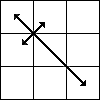F R U' R' U' R U R' F' R U R' U' R' F R F'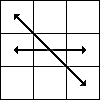R' U R U' R' F' U' F R U R' F R' F' R U' R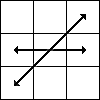F R U' R' U' R U R' F' R U R' U' R' F R F'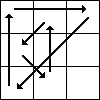y R2' u R' U R' U' R u' R2 y' R' U R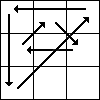R' U' R y R2 u R' U R U' R u' R2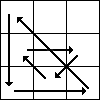y R2' u' R U' R U R' u R2 y R U' R'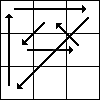y2 R U R' y' R2 u' R U' R' U R' u R2Cubezz.com - Professional Puzzle Store for Magic Cubes - Rubik's Cubes - Twisting Puzzles - Magic Cube Accessories
DaYan - MF8 - V-cube - Meffert's - SMAZ - WitEden - Lanlan - QJ - Shengshou - MoYu - Guojia - Mozhi -C4U - and More